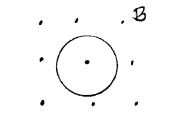# Problem: A circular loop of wire has raidus r = 0.20 m and resistance 40 Ω. The loop is in a uniform magnetic field that is directed out of the plane on the paper, as shown in the sketch. The magnetic field is decreasing at a constant rate of ΔB/Δt = -0.050 T/s. What are the magnitude and direction (clockwise or counterclockwise) of the current that is induced in the loop? Ans. I = Direction =

###### FREE Expert Solution
88% (475 ratings)
###### Problem Details

A circular loop of wire has raidus r = 0.20 m and resistance 40 Ω. The loop is in a uniform magnetic field that is directed out of the plane on the paper, as shown in the sketch. The magnetic field is decreasing at a constant rate of ΔB/Δt = -0.050 T/s. What are the magnitude and direction (clockwise or counterclockwise) of the current that is induced in the loop?

Ans. I =

Direction =